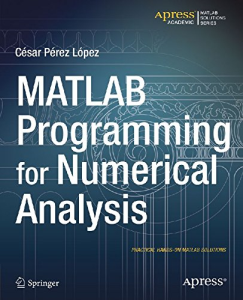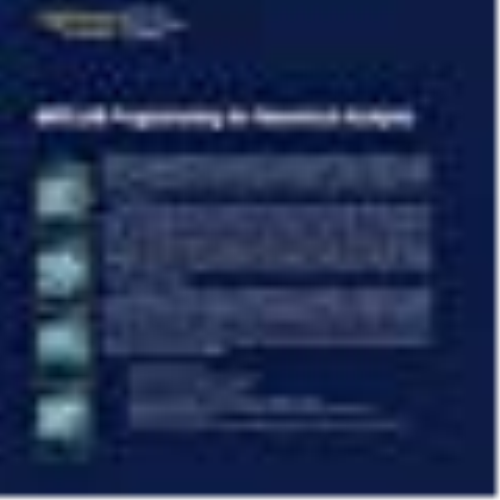# MATLAB Programming for Numerical Analysis (Matlab Solutions) (English Edition) 1st ed. Edition, Format Kindle

PLDZ-20 In Stock
\$ 5.00 USD
Description

MATLAB is a high-level language and environment for numerical computation, visualization, and programming. Using MATLAB, you can analyze data, develop algorithms, and create models and applications. The language, tools, and built-in math functions enable you to explore multiple approaches and reach a solution faster than with spreadsheets or traditional programming languages, such as C/C++ or Java.

Programming MATLAB for Numerical Analysis introduces you to the MATLAB language with practical hands-on instructions and results, allowing you to quickly achieve your goals. You will first become familiar with the MATLAB environment, and then you will begin to harness the power of MATLAB. You will learn the MATLAB language, starting with an introduction to variables, and how to manipulate numbers, vectors, matrices, arrays and character strings. You will learn about MATLAB’s high-precision capabilities, and how you can use MATLAB to solve problems, making use of arithmetic, relational and logical operators in combination with the common functions and operations of real and complex analysis and linear algebra.

You will learn to implement various numerical methods for optimization, interpolation and solving non-linear equations. You will discover how MATLAB can solve problems in differential and integral calculus, both numerically and symbolically, including techniques for solving ordinary and partial differential equations, and how to graph the solutions in brilliant high resolution. You will then expand your knowledge of the MATLAB language by learning how to use commands which enable you to investigate the convergence of sequences and series, and explore continuity and other analytical features of functions in one and several variables.

Recent Reviews Write a Review
0 0 0 0 reviews